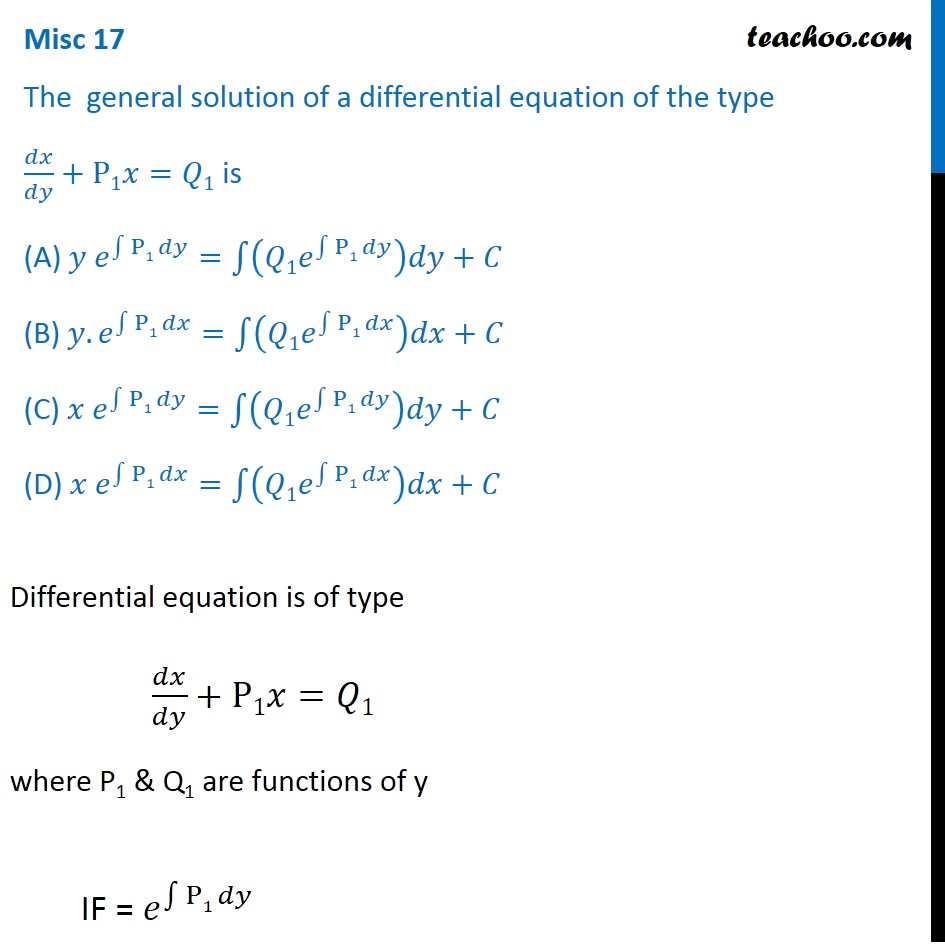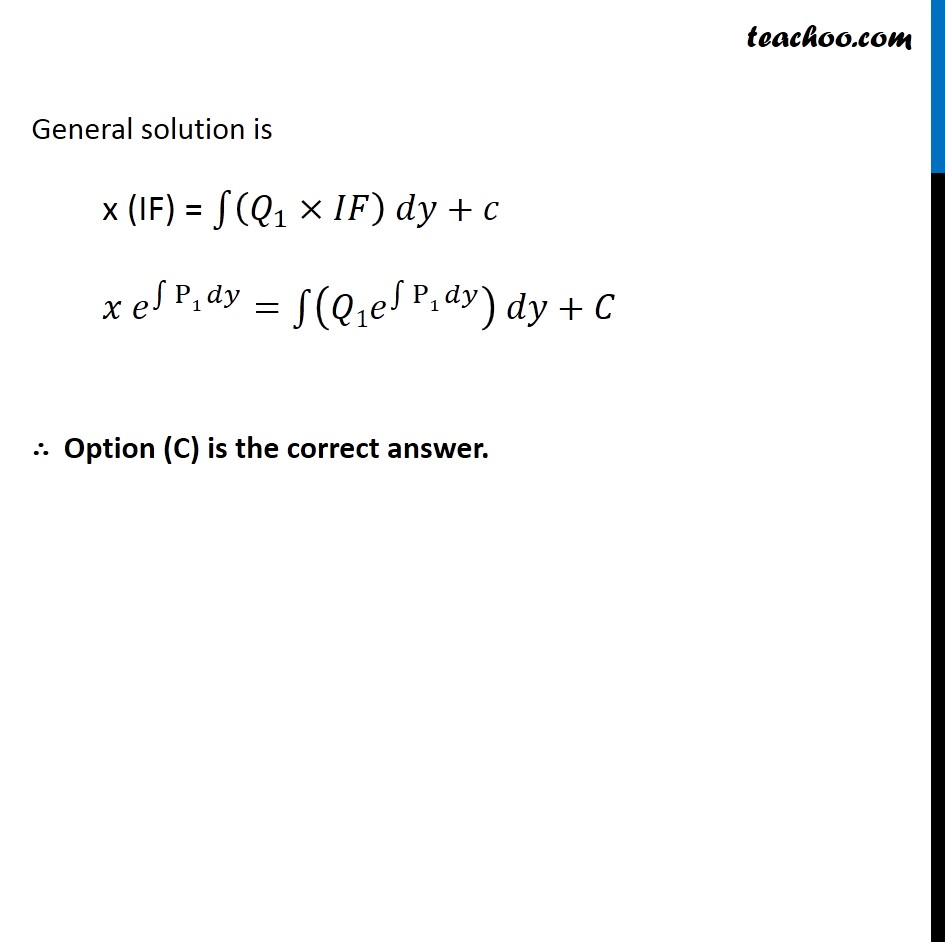Miscellaneous

Chapter 9 Class 12 Differential Equations
Serial order wiseLearn in your speed, with individual attention - Teachoo Maths 1-on-1 Class

### Transcript

Misc 17 The general solution of a differential equation of the type 𝑑𝑥/𝑑𝑦+P1𝑥=𝑄1 is (A) 𝑦 𝑒^∫1▒〖P1 𝑑𝑦〗=∫1▒〖(𝑄1𝑒^∫1▒〖P1 𝑑𝑦〗 )𝑑𝑦+𝐶〗 (B) 𝑦. 𝑒^∫1▒〖P1 𝑑𝑥〗=∫1▒〖(𝑄1𝑒^∫1▒〖P1 𝑑𝑥〗 )𝑑𝑥+𝐶〗 (C) 𝑥 𝑒^∫1▒〖P1 𝑑𝑦〗=∫1▒〖(𝑄1𝑒^∫1▒〖P1 𝑑𝑦〗 )𝑑𝑦+𝐶〗 (D) 𝑥 𝑒^∫1▒〖P1 𝑑𝑥〗=∫1▒〖(𝑄1𝑒^∫1▒〖P1 𝑑𝑥〗 )𝑑𝑥+𝐶〗 Differential equation is of type 𝑑𝑥/𝑑𝑦+P1𝑥=𝑄1 where P1 & Q1 are functions of y IF = 𝑒^∫1▒〖P1 𝑑𝑦〗 General solution is x (IF) = ∫1▒〖(𝑄_1×𝐼𝐹) 𝑑𝑦+𝑐〗 𝑥 𝑒^∫1▒〖P1 𝑑𝑦〗=∫1▒〖(𝑄1𝑒^∫1▒〖P1 𝑑𝑦〗 ) 𝑑𝑦+𝐶〗 ∴ Option (C) is the correct answer.